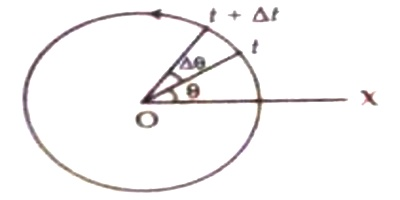Physics

# Angular VelocityAngular velocity

Angular velocity, also called rotational velocity, is a quantitative expression of the amount of rotation that a spinning object undergoes per unit time. Like linear motion, the angular motion can also be uniform or non-uniform. If the angular displacement is non-uniform the ratio of the angular displacement and the time interval for that displacement is called average angular velocity of the particle. It is denoted by ω. The angular velocity can either be positive or negative. The similar rule like angular displacement is applied here.If during exceedingly small time interval ∆t, the angular displacement of the particle is ∆θ (Fig), then average angular velocity for that time interval is,

ω = ∆θ/∆t

In order to know instantaneous velocity at a particular instant, the time interval is made smaller and smaller. If time interval tends to zero, the average angular velocity during that time interval is equal to instantaneous angular velocity. So, the rate of change of instantaneous angular displacement is called instantaneous angular velocity ω. That means,

ω = Lit∆t→0 (∆θ/∆t) = dθ/dt

In order to know instantaneous velocity at a particular instant, the time interval is made smaller and smaller. If time interval tends to zero, the average angular velocity during that time interval is equal to instantaneous angular velocity. So, the rate of change of instantaneous angular displacement is called instantaneous angular velocity ω.

That means;

ω = Lit∆t→0 (∆θ/∆t) = dθ/dt

Normally, angular velocity means instantaneous angular velocity.

If the angular velocity is constant, then that circular motion is called uniform circular motion. In case of uniform circular motion at time t the angular displacement is θ, the magnitude of angular velocity is,

ω = θ/t; or, θ = ωt

This equation is similar to the equation of uniform linear motion, s = vt.

Unit: Generally angular velocity is expressed in radian/sec or rad/s unit. In mechanical or engineering another unit is in use. It is called rotation or revolution per minute (rpm)

Dimension of angular velocity [ω] = [linear velocity / radius]

= [LT-1] / [L]

= [T-1]

Example: A race car on a circular track, a roulette ball on a roulette wheel or a Ferris wheel; all have an angular velocity.